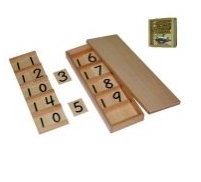ACTIVITY:

## Montessori Seguin Board A

MATERIALS:Sequin Board A

Number cards 1 – 9

A floor mat

OBJECTIVES:

To teach the child the symbols from 11 – 19.

CONTROL OF ERROR:

The three period lesson.

AGE: 4 – 5 years approx.
• Leave the card in place and continue with 12.
• Point to the next number 10 on the board and ask the child if he remembers what that number is.
• The child should respond with, “ten” in which case you continue the lesson.
• Show the child how to take the loose “two” number card and how to slide it into the right hand side of the board covering the 0 so that the number now formed is 12 which is directly below the 11.
• Remind the child that when we added two to the ten (as we did with the beads) we make a new number called twelve. This is how we would write 12.
• Repeat this with 13.
• In the SECOND PERIOD repeatedly ask the child to show you the various amounts 11, 12 and 13.
• In the THIRD PERIOD, try to isolate each amount by covering each top number formed with a small piece of felt. Asking the child (in order) “what number is this?”
• Consolidate by asking the child again to tell you the name of all three numbers.
• The next time you work with this, do one known and two unknown numbers.
• End the work cycle.
• Sorry, the comment form is closed at this time.

+ +